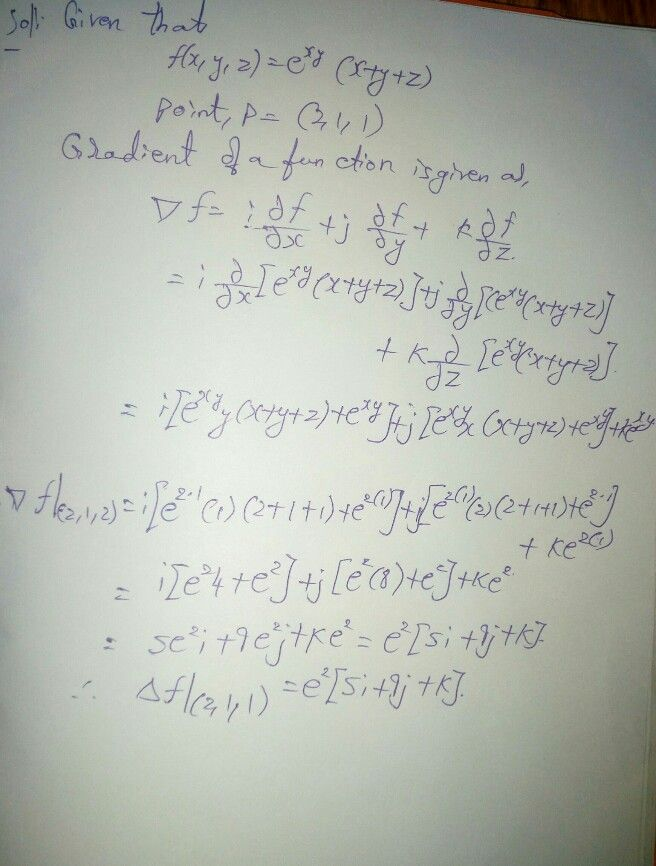Symbol
ProblemCompute the gradient of the scalar function f(x, $y,z\right)=xy$ $\left(x+y+z\right)$ $at\left(2,1,1\right)$ $4^{\right)}0>$
Algebra
Search count: 110
SolutionQanda teacher - aakarcheck the solution plseStudent
thank you very much, best answer and understandable